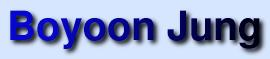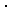Boyoon Jung's Library Manual

# bj::KDCluster Class Reference [Machine Learning Techniques]

A fast data clustering algorithm using a kernel density tree. More...

`#include <kd_cluster.h>`

List of all members.

## Public Member Functions

KDCluster (int dimension, double threshold, int max_clusters=10)
A constructor.
~KDCluster (void)
A destructor.
virtual int cluster (KDTree *tree)
Cluster data points using multiple gaussians.
int numClusters (void)
Return the number clusters.
gsl_vector * mean (int c)
Return the mean vector of a specific cluster.
gsl_matrix * covariance (int c)
Return the covariance matrix of a specific cluster.
double densityThreshold (void)
Return the density threshold.
void setDensityThreshold (double th)
Set a new density threshold.

## Static Public Member Functions

void cov2ellipse (gsl_matrix *cov, double &w, double &h, double &a)
Convert a covariance matrix to an ellipsoidal parameters.

## Protected Member Functions

double distance (gsl_vector *x, gsl_vector *y)
Compute the manhattan distance between two nodes.
void select_candidates (kd_node *tree, int npoints, kd_node **candidates, int &size)
Select candidate nodes from a kd-tree.

## Protected Attributes

int nclusters
Number of clusters.
gsl_vector ** m
Mean vectors.
gsl_matrix ** cov
Covariance matrices.
int dimension
The dimension of input points.
int max_clusters
Maximum number of clusters.
double threshold
Density threshold.
gsl_vector * offsetv
Offset of a mean vector.
gsl_vector * mv
New mean vector.
gsl_vector * nmv
Newly introduced mean vector.
gsl_matrix * ncm
Newly introduced covariance matrix.
gsl_vector * tmpv
Temporary buffer.
gsl_matrix * tmpm
Temporary buffer.

## Detailed Description

A fast data clustering algorithm using a kernel density tree.

KDCluster class implements a fast data clustering algorithm using a KD-tree. The clustering result is multiple, independent gaussians.

This class is mainly for clustering particles generated by AdaptiveParticleFilter class. It takes a kd-tree as an input instead of building one internally because AdaptiveParticleFilter class build a tree anyway to compute a proper number of particles.

## Constructor & Destructor Documentation

 bj::KDCluster::KDCluster ( int dimension, double threshold, int max_clusters = `10` )

A constructor.

Parameters:
 dimension The dimension of data points. threshold The density threshold. max_clusters The maximum number of clusters.

 bj::KDCluster::~KDCluster ( void )
 A destructor.

## Member Function Documentation

 virtual int bj::KDCluster::cluster ( KDTree * tree ) ` [virtual]`
 Cluster data points using multiple gaussians. Returns:The number of clusters.

 void bj::KDCluster::cov2ellipse ( gsl_matrix * cov, double & w, double & h, double & a ) ` [inline, static]`

Convert a covariance matrix to an ellipsoidal parameters.

Parameters:
 cov A covariance matrix. [out] w The width of an ellipsoidal. [out] h The height of an ellipsoidal. [out] a The angle (in radians) of an ellipsoidal.

 gsl_matrix* bj::KDCluster::covariance ( int c ) ` [inline]`
 Return the covariance matrix of a specific cluster.

 double bj::KDCluster::densityThreshold ( void ) ` [inline]`
 Return the density threshold.

 double bj::KDCluster::distance ( gsl_vector * x, gsl_vector * y ) ` [inline, protected]`
 Compute the manhattan distance between two nodes.

 gsl_vector* bj::KDCluster::mean ( int c ) ` [inline]`
 Return the mean vector of a specific cluster.

 int bj::KDCluster::numClusters ( void ) ` [inline]`
 Return the number clusters.

 void bj::KDCluster::select_candidates ( kd_node * tree, int npoints, kd_node ** candidates, int & size ) ` [protected]`
 Select candidate nodes from a kd-tree.

 void bj::KDCluster::setDensityThreshold ( double th ) ` [inline]`
 Set a new density threshold.

## Member Data Documentation

 gsl_matrix** bj::KDCluster::cov` [protected]`
 Covariance matrices.

 int bj::KDCluster::dimension` [protected]`
 The dimension of input points.

 gsl_vector** bj::KDCluster::m` [protected]`
 Mean vectors.

 int bj::KDCluster::max_clusters` [protected]`
 Maximum number of clusters.

 gsl_vector* bj::KDCluster::mv` [protected]`
 New mean vector.

 int bj::KDCluster::nclusters` [protected]`
 Number of clusters.

 gsl_matrix* bj::KDCluster::ncm` [protected]`
 Newly introduced covariance matrix.

 gsl_vector* bj::KDCluster::nmv` [protected]`
 Newly introduced mean vector.

 gsl_vector* bj::KDCluster::offsetv` [protected]`
 Offset of a mean vector.

 double bj::KDCluster::threshold` [protected]`
 Density threshold.

 gsl_matrix* bj::KDCluster::tmpm` [protected]`
 Temporary buffer.

 gsl_vector* bj::KDCluster::tmpv` [protected]`
 Temporary buffer.

The documentation for this class was generated from the following file:
ResearchProjectsPublications

ToolsBjLib ManualPlayer/StagePyPlayerMicroStrain IMUGPS/DGPSParticle Filter

PersonalWelcomeCurriculum VitaeResumeContact

Outside LinksUSCUSC CS DeptCRESRobotics LabRESL